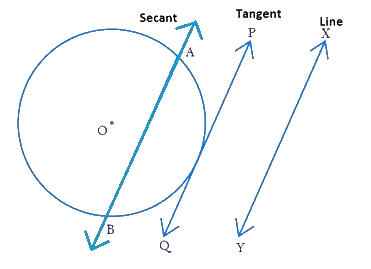# Ex.10.1 Q2. CIRCLES Solution - NCERT Maths Class 10

Go back to  'Ex.10.1'

## Question

Fill in the blanks:

(i) A tangent to a circle intersects it in _________ point (s).

(ii) A line intersecting a circle in two points is called a ____________.

(iii) A circle can have _________ parallel tangents at the most.

(iv) The common point of a tangent to a circle and the circle is called _________ .

Video Solution
Circles
Ex 10.1 | Question 2

## Text Solution

Steps:

(i) A tangent to a circle intersects it in       One         point (s).

Reasoning:

A tangent to a circle is a line that intersects the circle at only one point.

(ii) A line intersecting a circle in two points is called a    Secant   .

Reasoning:

Secant is a line that intersects the circle in two points.(iii) A circle can have    Two     parallel tangents at the most.

Reasoning:

Tangent at any point of a circle is perpendicular to the radius through the point of contact. Extended radius is a diameter which has two end points and hence two tangents which are parallel to themselves and perpendicular to the diameter.

Center $$O,$$ diameter $$AB,\text{ tangents}\, PQ, RS$$ and, $${PQ}\; \| \;{RS}$$

$$A$$ and $$B$$ are called as point of contact.

(iv) The common point of a tangent to a circle and the circle is called    Point of contact     .

Reasoning:

A tangent to a circle is a line that intersects the circle at only one point and that point is called as point of contact.

Learn from the best math teachers and top your exams

• Live one on one classroom and doubt clearing
• Practice worksheets in and after class for conceptual clarity
• Personalized curriculum to keep up with school